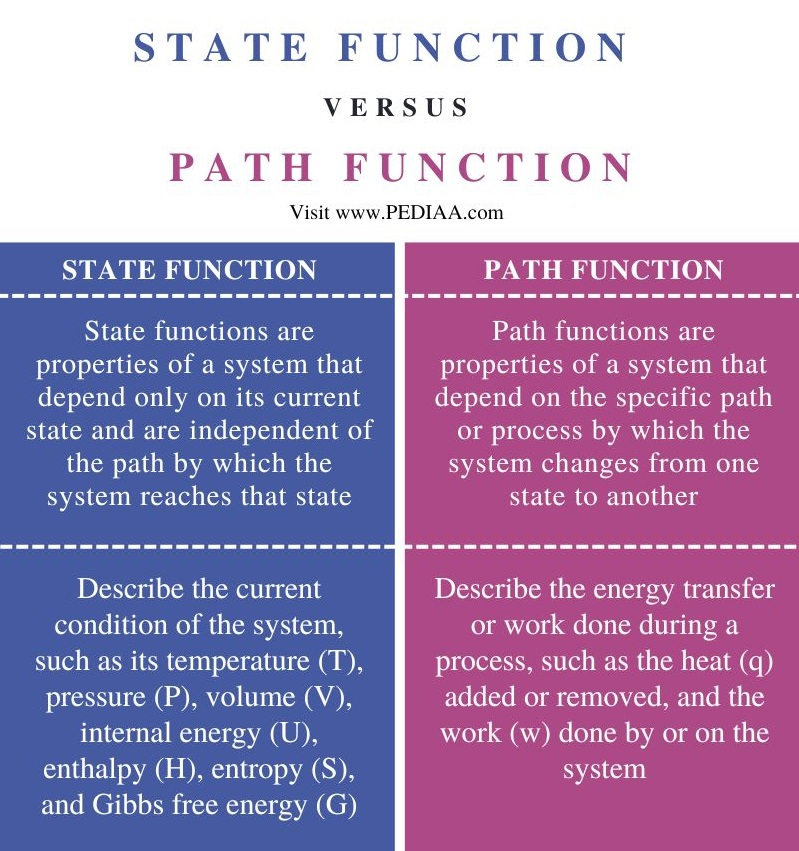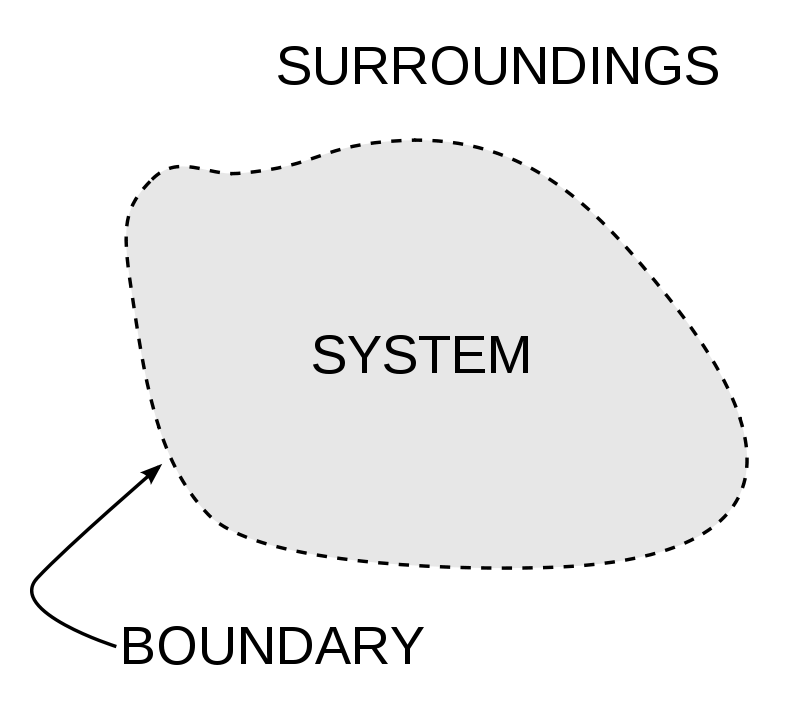# What is the Difference Between State Function and Path Function

The main difference between state function and path function is that state function describes the overall state of a system and is path-independent, while path functions relate to specific processes and are path-dependent.

State functions and path functions are concepts used in thermodynamics to describe how a system behaves and how energy is transferred within the system. They are important for understanding and analyzing the thermodynamic properties of substances and processes.

### Key Areas Covered

1. What is a State Function
– Definition, Features
2. What is a Path Function
– Definition, Features
3. Similarities Between State Function and Path Function
– Outline of Common Features
4. Difference Between State Function and Path Function
– Comparison of Key Differences

### Key Terms

State Function, Path Function## What is a State Function

In thermodynamics, a state function is a fundamental concept used to describe the properties of a system. State functions are quantities that depend only on a system’s current state or condition, irrespective of how that state was reached. The state variables of the system define them and are often used to describe the equilibrium state of a system.

The key idea behind state functions is that they capture the intrinsic characteristics of a system at a specific moment. These characteristics are independent of the path taken to reach that state. In other words, the values of state functions depend solely on the initial and final states of the system, not on the specific process or pathway connecting those states. This path-independence makes state functions valuable tools in the field of thermodynamics. Examples of state functions include internal energy, temperature, pressure, volume, and enthalpy.

The fact that state functions are independent of the specific processes that occur allows for the simplification of thermodynamic calculations. It means that one can often determine the change in a system’s state without needing to know all the intermediate steps of a process. State functions are particularly relevant when studying systems in thermodynamic equilibrium. Equilibrium is a state where all state functions have reached their stable values, making it possible to describe and predict the behavior of systems under various conditions. State functions play a central role in formulating fundamental thermodynamic laws, such as the first law (conservation of energy) and the second law (entropy increases in isolated systems). These laws are expressed in terms of state functions.Figure 1: A Basic System in Thermodynamics

### Applications of Path Function

State functions find application in various areas of science and engineering, including. In chemistry, state functions like enthalpy (ΔH) and Gibbs free energy (ΔG) help to determine whether a chemical reaction is spontaneous or requires an input of energy. They also help in predicting reaction outcomes under different conditions. Engineers use state functions to analyze and design thermodynamic processes; for example, designs of heat engines, refrigeration cycles, and phase transitions. In materials science, state functions are crucial for understanding phase diagrams, phase transitions, and the behavior of materials under varying conditions of temperature and pressure. Moreover, state functions are applied to study biological systems, such as metabolic pathways and enzyme kinetics. In addition, state functions help to assess the thermodynamics of environmental processes, including the behavior of pollutants, chemical reactions in natural systems, and energy transformations in ecosystems.

## What is a Path Function

Path functions are system properties that depend on the specific pathway or process taken to reach a particular state from another. Unlike state functions, path functions characterize the journey itself. They describe how energy is transferred or work is done during a process. Path functions depend on the specific conditions, the sequence of events, and the intermediate states experienced during the process.

The term “path function” is derived from the fact that these properties are contingent on the path or route a system follows as it transitions from one state to another. Several common thermodynamic properties are classified as path functions. Some notable examples include work, heat, and path-dependent energies. Path functions, particularly work and heat, allow for a detailed accounting of energy changes during physical and chemical processes. They help assess how energy is transferred between a system and its surroundings.

Path functions provide a deeper understanding of the mechanics of a process, including the forces, pressures, and temperature changes involved. This insight is essential in designing and optimizing various engineering processes. Work (W) as a path function is fundamental in analysing mechanical systems, such as engines, turbines, and pumps. It helps quantify the energy required for or produced by these devices. Heat as a path function is vital in understanding heat exchangers, refrigeration cycles, and various thermal systems. It facilitates calculating energy transfers through conduction, convection, and radiation.

## Similarities Between State Function and Path Function

• State functions and path functions are both used in thermodynamics to describe and analyze the behavior of systems and the transfer of energy within those systems.
• Both functions are integral to the analysis of energy changes within a system.

## Difference Between State Function and Path Function

### Definition

State functions are properties of a system that depend only on its current state and are independent of the path by which the system reaches that state, while path functions are properties of a system that depend on the specific path or process by which the system changes from one state to another.

### Examples

State functions describe the current condition of the system, such as its temperature (T), pressure (P), volume (V), internal energy (U), enthalpy (H), entropy (S), and Gibbs free energy (G), whereas path functions describe the energy transfer or work done during a process, such as the heat (q) added or removed, and the work (w) done by or on the system.

## Conclusion

In brief, state functions and path functions are concepts in thermodynamics that describe the behavior and the energy transfer in a system. The main difference between state function and path function is that state function describes the overall state of a system and is path-independent, while path functions relate to specific processes and are path-dependent.

##### Reference:

1. “State Function.” Wikipedia. Wikipedia Foundation.

##### Image Courtesy:

1. “System boundary” By Original: Wavesmikey / Vectorization: Stannered – Own work based on: System-boundary.jpg (Public Domain) via Commons Wikimedia### About the Author: Hasini A

Hasini is a graduate of Applied Science with a strong background in forestry, environmental science, chemistry, and management science. She is an amateur photographer with a keen interest in exploring the wonders of nature and science.